# MAX Function

Returns the largest value in a given list of arguments

## What is the MAX Function?

The MAX Function is categorized under Excel Statistical functions. MAX will return the largest value in a given list of arguments. From a given set of numeric values, it will return the highest value. Unlike MAXA function, the MAX function will count numbers but ignore empty cells, text, the logical values TRUE and FALSE, and text values.

In financial analysis, MAX can be useful in calculating the highest score, the fastest time, the highest expense or revenue amount, etc.

### Formula

=MAX(number1, [number2], …)

Number1 and number2 are the arguments used for the function, where Number1 is required and the subsequent values are optional.

In MS Excel 2007 and later versions, we can provide up to 255 number arguments to the MAX function. However, in Excel 2003 and earlier versions, it can only accept up to 30 number arguments.

Arguments can be provided as constants, or as cell references or ranges. If an argument is supplied to the function as a reference to a cell, or an array of cells, the MAX function will ignore blank cells, and text or logical values contained within the supplied cell range. However, logical values and text representations of numbers that are supplied directly to the function will be included in the calculation.

### How to use the MAX Function in Excel?

As a worksheet function, the MAX function can be entered as part of a formula in a cell of a worksheet. To understand the uses of the function, let us consider an example:

#### Example

Let us calculate the highest marks from the following data: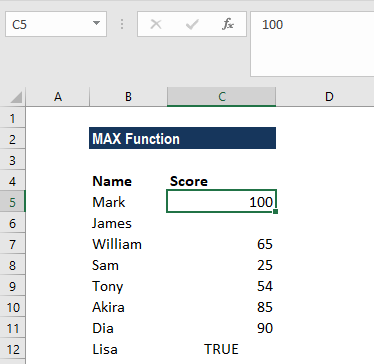The formula used was: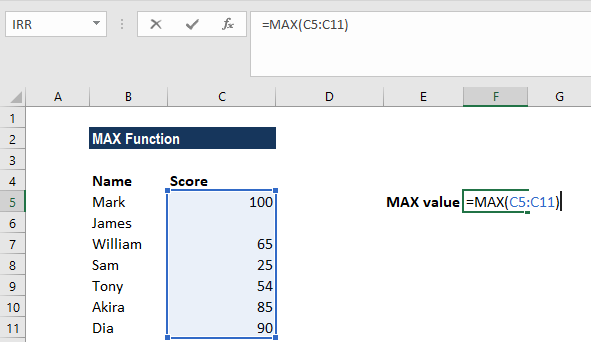The MAX function ignored the empty value and returned 100 as the result.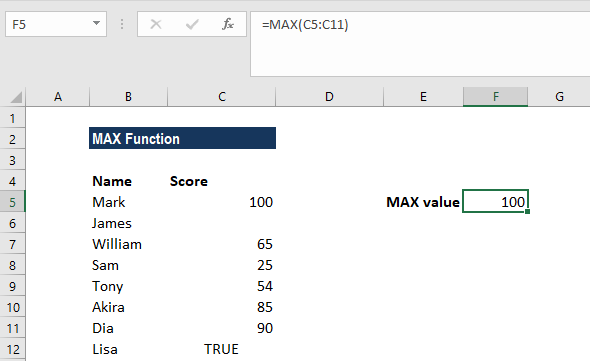As seen above, MAX will ignore empty values. In this example, if we provide a logical value, the function will ignore it and give the same result, as shown below: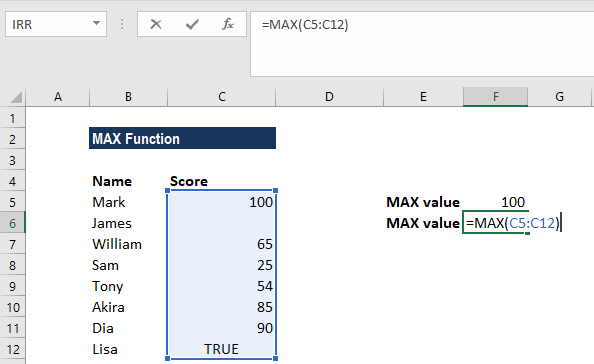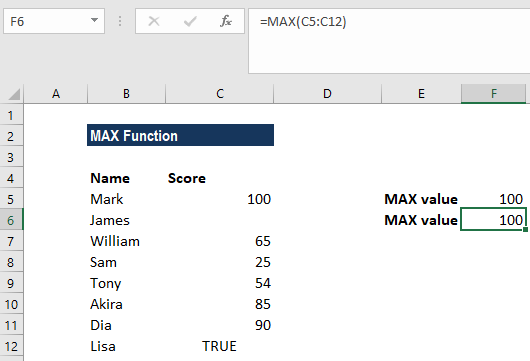### A few things to remember about the MAX Function

1. #VALUE! error – Occurs if any values that are provided directly to the MAX function are non-numeric.
2. If the arguments contain no numbers, MAX returns 0.
3. The primary difference between MAX and MAXA is that MAXA evaluates TRUE and FALSE values as 1 and 0, respectively. Hence, if we wish to include logical values, then we need to use the MAXA function.

Thanks for reading CFI’s guide to important Excel functions! By taking the time to learn and master these functions, you’ll significantly speed up your financial modeling. To learn more, check out these additional CFI resources:

• Excel Functions for Finance